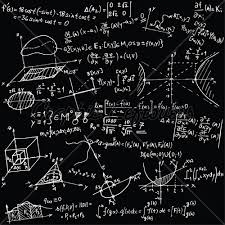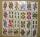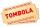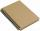# Year 2020

The four-digit number divided by 2020 gives a result of 1, **. (Can not be in form 1,*0. ) Write all the options.

Correct result:

n1 =  2323
n2 =  2525
n3 =  2727
n4 =  2929
n5 =  3131
n6 =  3333
n7 =  3535
n8 =  3737
n9 =  3939

#### Solution:Our examples were largely sent or created by pupils and students themselves. Therefore, we would be pleased if you could send us any errors you found, spelling mistakes, or rephasing the example. Thank you!

Please write to us with your comment on the math problem or ask something. Thank you for helping each other - students, teachers, parents, and problem authors.Tips to related online calculators
Do you want to perform natural numbers division - find the quotient and remainder?
Would you like to compute count of combinations?

## Next similar math problems:

• Three workplacesHow many ways can we divide nine workers into three workplaces if they need four workers in the first workplace, 3 in the second workplace and 2 in the third?
• ClassroomOf the 26 pupils in the classroom, 12 boys and 14 girls, four representatives are picked to the odds of being: a) all the girls b) three girls and one boy c) there will be at least two boysThere are 15 boys and 12 girls at the graduation party. Determine how many four couples can be selected.
• Two groupsThe group of 10 girls should be divided into two groups with at least 4 girls in each group. How many ways can this be done?
• STRESSED wordEach letter in STRESSED is printed on identical cards, one letter per card and assembled in random order. Calculate the probability that the cards spell DESSERTS when assembled.
• SalamiHow many ways can we choose 5 pcs of salami if we have 6 types of salami for 10 pieces and one type for 4 pieces?How many ways can we thread 4 red, 5 blue, and 6 yellow beads onto a thread?
• Boys and girlsThere are 11 boys and 18 girls in the classroom. Three pupils will answer. What is the probability that two boys will be among them?
• Families 2There are 729 families having 6 children each. The probability of a girl is 1/3 and the probability of a boy is 2/3. Find the the number of families having 2 girls and 4 boys.
• Sum or productWhat is the probability that two dice fall will have the sum 7 or product 12?
• Three redsWhat is the probability that when choosing 3 carats from seven carats, all 3 reds will be red?
• CardsFrom a set of 32 cards we randomly pull out three cards. What is the probability that it will be seven king and ace?
• Combinations of sweatersI have 4 sweaters two are white, 1 red and 1 green. How many ways can this done?
• Boys and girlsThere are eight boys and nine girls in the class. There were six children on the trip from this class. What is the probability that left a) only boys b) just two boys
• RaffleThere are 200 draws in the raffle, but only 20 of them win. What is the probability of at least 4 winnings for a group of people who have bought 5 tickets together?
• TournamentSix teams entered the basketball tournament. How many matches will be played if each team has to play one match with each other?
• Word MATEMATIKAHow many words can be created from the word MATEMATIKA by changing the order of the letters, regardless of whether or not the words are meaningful?